SCHOLASTIC APTITUDE TEST 1998

CHEMISTRY

 Time: 1 Hour Max.Marks : 60

Instructions:

• Answer must be written either in English or the medium of instruction of the candidate in high school.
• There will be no negative marking
• Use of calculators or graph papers is not permitted
• There are SIX Questions. Answer all the questions.

I. Multiple choice. Each question has one or more than one answer(s). Indicate the correct answer(s) by A,B,C,D. (5x1 = 5 Marks)

1.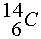is a beta-active nucleus. A sample 14CH4 gas kept in a closed vessel shows increase in pressure with time. This is due to

 A) formation of 14NH3 and H2 B) formation of 11BH3 and He C) formation of 14C2H4 and H2 D) formation of 12CH3, 14NH2 and H2

2. For a particular value of azimuthal quantum number (l) the total number of magnetic quantum number (m) is given by

 A)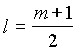B)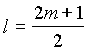C)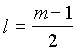D)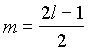3. For a reaction 4A+B ® 2C+2D

which of the following statements is/are not correct

A) The rate of disappearance of B is one forth the rate of disappearance of A

B) Rate of appearance of C is one-half the rate of disappearance of B

C) The rate of formation of D is one-half the rate of consumption of A

D) Rate of formation of D is one-half the rate of consumption of A

4. Choose the correct statements

A) Under the similar conditions of pressure and temperature one mole of

all the gases have the same volumes

B) The average K.E. of gas molecules is proportional to absolute temperature

C) At absolute zero temperature the volume of a gas is expected to be zero

D) 2730C and 760 mm of Hg pressure are the standard temperature and pressure

5. Five valance electrons of 15P are labelled as

If spin quantum of B and Z is +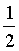.

The group of electrons with three of the quantum number same are

 A) XYZ, AZ B) AB, XYZ, BY C) AB D) AB, XYZ

 II. Write the balanced Chemical Equations for following chemical changes (5x2=10 Marks)

1. Elemental phosphorus reacts with conc.HNO3.

2. Carbondioxide is passed through a suspension of lime stone in water.

3. Conc.H2SO4 is drop wise added to the mixture of NaCl and MnO2.

4. NaCl reacts with an aqueous solution of NH3 saturated with CO2 gas.

5. Aqueous solution containing potassium nitrate and ferrous sulphate is treated with conc.H2SO4.

III. Give reasons in one or two sentences for the following: (10x2 = 20 Marks)

(Write the chemical equations wherever necessary)

1. The hydroxides of aluminium and Iron are insoluble in water. However, NaOH is used to separate one from the other.

2. Helium is monatomic, while hydrogen is diatomic molecule.

3. Fluorine has less electron affinity than chlorine but electronegativity of fluorine is more than that of chlorine.

4. White precipitate is formed when AgNO3 is treated with NaCl(aq) but no precipitate is formed when AgNO3 is treated with CCl4.

5. Dry SO2 and Cl2 does not bleach dry flowers.

6. Although aluminium is above hydrogen in the electrochemical series it is stable in air and water.

7. When hydrogen gas is bubbled through acidified potassium permanganate solution, pink colour is not discharged, but when few pieces of metal zinc are added to it, the pink colour disappears immediately.

8. When blue crystals of CuSO4.5H2O are added to conc.H2SO4 they turn white.

9. Ferric chloride solution used as a reagent in the laboratory is prepared by dissolving, Ferric chloride not in mere water, but in hydrochloric acid.

10. Sulphur melts to a clear mobile liquid at 1190C but on further heating above 160oC it becomes viscous.

 IV. Fill in the blanks (15x1 = 15 Marks)

1. The weight of 1x1022 molecules of CuSO4.5H2O is ________________.

(atomic weight of Cu=63.5; S=32; O=16 amu)

2. The blue complex formed, on addition of conc.NH4OH solution to a Cu2+ salt solution has the structure _________________.

3. The temperature at which the real gases obey the equation pV=nRT under all pressure conditions is known as ___________.

4. Molten sodium chloride conducts electric current due to the presence of __________.

5. The atomicity of a species is x and its atomic weight is y. The molecular weight of the species is ___________________.

6. An element has atomic weight 2Z+6 where Z is the number of extra nuclear electrons in the atom, then the number of neutrons in the nucleus is ____________.

7. A container holds an equimolar mixture of H2 and O2. If through small hole the mixture is allowed to diffuse, the mass ratio of H2:O2 in the initial diffusing gases is ___________.

8. Helium obtained at STP by the disintegration of 2 grams of 224Ra after 3.65 days in a closed vessel t1/2 of 224Ra is 3.65 days _____________.

9. The shift in the equilibrium 2CO + O2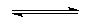2CO2 + Heat

on increasing the temperature and decreasing the volume simultaneously, is __________.

10. Equal weights of hydrogen and methane are mixed in an empty container at 250C. The fraction of total pressure exerted by hydrogen is ___________________.

11. An element which can exist as a positive in acidic solution and also as a negative ion in basic solution is said to be ____________.

12. A mixture of hydrogen and oxygen (45 ml) is sparked to form liquid water. The component not in excess reacts completely and 15 ml is leftover (all measurements are at the same temperature and pressure). The composition by volume in the original mixture of H2 and O2 is ____________.

13. The formula of deep red liquid formed on warming potassium dichromate with KCl in concentrated sulphuric acid is _______________.

14. The metal which reacts with dilute nitric acid but becomes passive with conc. Nitric acid is _______________.

15. Ship hull is protected against corrosion by attaching zinc blocks because ___________.

V. Arrange the following as stated (5x1 = 5 Marks)

a) Increasing order of ionic size

[N3- , Na+ , F-, O2-, Mg2+ ]

b) Increasing the strength of hydrogen bonding (X ……… H - X )

[O, S, F, Cl, N]

c) Increasing order of basic character

[MgO, SrO, K2O, NiO, Cs2O]

d) Increasing order of bond dissociation

[N2, O2, F2, Cl2]

e) Increasing order of Ist I.P.

[Na, Al, Mg, Si]

VI. Explain the following: (5x1 = 5 Marks)

(Give the Chemical equations wherever required)

1. What do you mean by Hardwater , heavy water, and distilled water.

2. Name the element whose atomic number is 17 and atomic mass is 35.5. Explain why it has fractional atomic mass.

3. State and explain the principle which restricts the number of electrons in an orbital to two.

4. HCl can be prepared by heating NaCl with conc.H2SO4while HBr cannot be prepared by heating NaBr with conc.H2SO4. Explain why?

5. Magnesium metal burns in air to give a white ash. When this ash is treated with water the odour of ammonia can be detected. What is the reason.

HOME